### Home > A2C > Chapter 13 > Lesson 13.1.1 > Problem13-21

13-21.
1. Change the angle measures below from radians to degrees or degrees to radians. Homework Help ✎

1. 108°

2. 320°

3.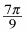4.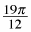5.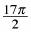6. 260°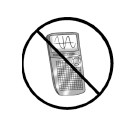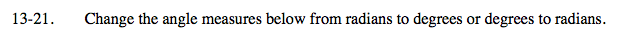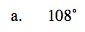$108\degree \cdot \frac{\pi}{180\degree}=\frac{3\pi}{5}$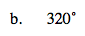See part (a).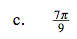$\frac{7\pi}{9}\cdot\frac{180\degree}{\pi}=140\degree$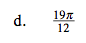See part (c).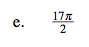See part (c).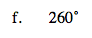See part (a).